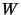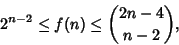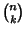## Convex Polygon

A Polygon is Convex if it contains all the Line Segments connecting any pair of its points. Letbe the smallest number such that whenis a set of more thanpoints in General Position (with no three points Collinear) in the plane, all of the Vertices of some convex-gon are contained in. The answers for, 3, and 4 are 2, 4, and 8. It is conjectured that, but only proven thatwhereis a Binomial Coefficient.